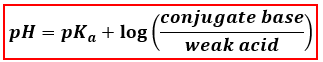# Problem: What is the pH of a buffer solution that is 0.24 M NH 3 and 0.20 M NH4Cl?

###### FREE Expert Solution

We’re being asked to calculate the pH of a solution the contains 0.24 M NH 3 and 0.20 M NH4Cl.

A solution that contains a weak base and its conjugate acid is a buffer solution. The pH of a buffer solution can be determined using the Henderson-Hasselbalch equation.Given solution:

The solution is composed of 0.24 M NH 3 and 0.20 M NH4Cl.

NH 3  is a weak base
the cation in NH4Cl is the conjugate acid

NH4Cl → NH4+ + Cl-

100% (381 ratings)###### Problem Details

What is the pH of a buffer solution that is 0.24 M NH 3 and 0.20 M NH4Cl?